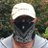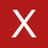The linear cross-entropy benchmark (Linear XEB) has been used as a test for procedures simulating quantum circuits. Given a quantum circuit $C$ with $n$ inputs and outputs and purported simulator whose output is distributed according to a distribution $p$ over $\{0,1\}^n$, the linear XEB fidelity of the simulator is $\mathcal{F}_{C}(p) = 2^n \mathbb{E}_{x \sim p} q_C(x) -1$ where $q_C(x)$ is the probability that $x$ is output from the distribution $C|0^n\rangle$. A trivial simulator (e.g., the uniform distribution) satisfies $\mathcal{F}_C(p)=0$, while Google's noisy quantum simulation of a 53 qubit circuit $C$ achieved a fidelity value of $(2.24\pm0.21)\times10^{-3}$ (Arute et. al., Nature'19). In this work we give a classical randomized algorithm that for a given circuit $C$ of depth $d$ with Haar random 2-qubit gates achieves in expectation a fidelity value of $Ω(\tfrac{n}{L} \cdot 15^{-d})$ in running time $\textsf{poly}(n,2^L)$. Here $L$ is the size of the \emph{light cone} of \$

5 mentions:Date: 2020/05/07 15:50

## Referring Tweets@TomoyukiMorimae t.co/SxQWXNG2r4 今日出た、クロスエントロピーベンチマークをSpoofingする話。ライトコーンのdisjointな量子ビットのいくつかについては確率分布を古典計算し、それ以外は一様分布を出す、という面白いアイデア。@sevag_gharibian Interesting paper today by Barak, Chou and Gao on classical algorithms which do well against the linear cross entropy benchmark used by the Google team for their quantum supremacy experiment t.co/fVdI0wKBAJ

## Related Entries[2002.05227] Variational Autoencoders with Riemannian Brownian Motion Priorscontact arXivarXiv Twitt...
0 users, 1 mentions 2020/02/15 00:51[2004.10809] Polarized-VAE: Proximity Based Disentangled Representation Learning for Text Generation...
0 users, 1 mentions 2020/04/24 21:51